# Pell equation

(diff) ← Older revision | Latest revision (diff) | Newer revision → (diff)

A Diophantine equation (cf. Diophantine equations) of the form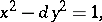(1)

as well as the more general equation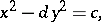(2)

whereis a positive integer,is an irrational number,is an integer, and the unknownsandare integers.

If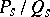,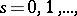are the convergent fractions for the expansion of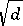in a continued fraction with period, then the positive solutions to (1) take the form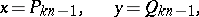whereis any natural number such thatis even.

All the solutions to (1) are derived from the formula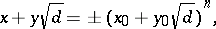whereis any integer and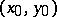is the solution with the least positive values for the unknowns. The general equation (2) either has no solutions at all or has infinitely many. For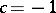, solutions exist if and only ifis odd. For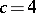, (2) always has solutions. The solutions to the Pell equation for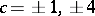are used in determining the units of the quadratic field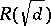. The solutions to a Pell equation are used to determine automorphisms of a binary quadratic form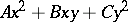; these enable one to use one solution to the Diophantine equation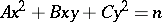to obtain an infinite set of solutions.

Equation (1) was examined by W. Brouncker (1657), P. Fermat and J. Wallis. L. Euler, on account of a misunderstanding, ascribed it to J. Pell.

How to Cite This Entry:
Pell equation. Encyclopedia of Mathematics. URL: http://encyclopediaofmath.org/index.php?title=Pell_equation&oldid=11604
This article was adapted from an original article by A.A. Bukhshtab (originator), which appeared in Encyclopedia of Mathematics - ISBN 1402006098. See original article